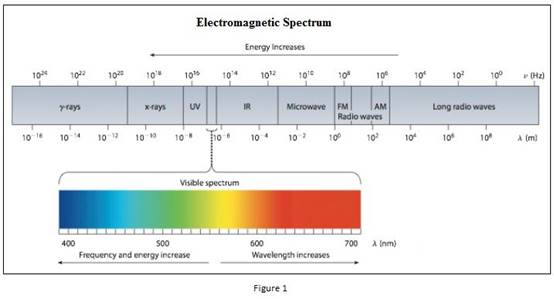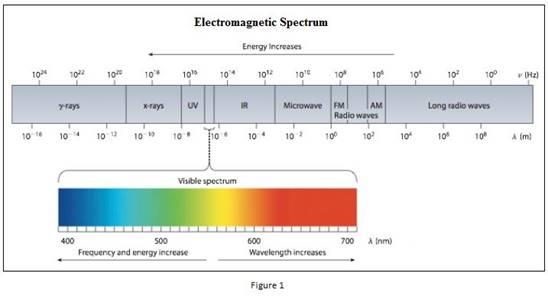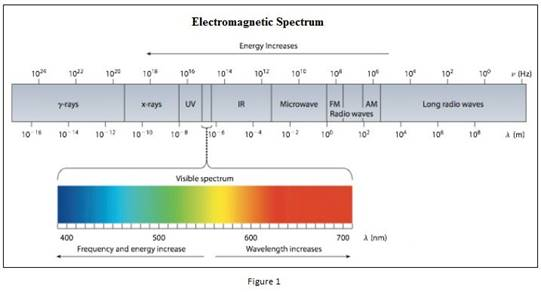# An advertising sign gives off red light and green light. (a) Which light has higher-energy photons? (b) One of the colors has a wavelength of 680 nm, and the other has a wavelength of 500 nm. Which color has which wavelength? (c) Which light has the higher frequency?### Chemistry & Chemical Reactivity

9th Edition
John C. Kotz + 3 others
Publisher: Cengage Learning
ISBN: 9781133949640

#### Solutions

Chapter
Section### Chemistry & Chemical Reactivity

9th Edition
John C. Kotz + 3 others
Publisher: Cengage Learning
ISBN: 9781133949640
Chapter 6, Problem 55GQ
Textbook Problem
60 views

## An advertising sign gives off red light and green light. (a) Which light has higher-energy photons? (b) One of the colors has a wavelength of 680 nm, and the other has a wavelength of 500 nm. Which color has which wavelength? (c) Which light has the higher frequency?

(a)

Interpretation Introduction

Interpretation: Among green light and red light, the colour having the higher energy photons should be identified.

Concept introduction:

• Electromagnetic radiations are a type of energy surrounding us. They are of different types like radio waves, IR, UV, X-ray etc.• The red light and green light lies in the visible light where it is between 400nm and 700nm.
• Planck’s equation,

E==hcλwhere, E=energyh=Planck'sconstantν=frequency

The energy increases as the wavelength of the light decrease. Also the energy increases as the frequency of the light increases.

• The frequency of the light is inversely proportional to its wavelength.

ν=cλwhere, c=speedoflightν=frequencyλ=wavelength

### Explanation of Solution

E=hcλ so the energy increases as the wavelength of the light decrease

(b)

Interpretation Introduction

Interpretation: Among green light and red light, the colour having 500nm and 680nm wavelength should be identified.

Concept introduction:

• Electromagnetic radiations are a type of energy surrounding us. They are of different types like radio waves, IR, UV, X-ray etc.• The red light and green light lies in the visible light where it is between 400nm and 700nm.

(c)

Interpretation Introduction

Interpretation: Among green light and red light, the colour having higher frequency should be identified.

Concept introduction:

• Electromagnetic radiations are a type of energy surrounding us. They are of different types like radio waves, IR, UV, X-ray etc.• The red light and green light lies in the visible light where it is between 400nm and 700nm.
• The frequency of the light is inversely proportional to its wavelength.

ν=cλwhere, c=speedoflightν=frequencyλ=wavelength

### Still sussing out bartleby?

Check out a sample textbook solution.

See a sample solution

#### The Solution to Your Study Problems

Bartleby provides explanations to thousands of textbook problems written by our experts, many with advanced degrees!

Get Started

Find more solutions based on key concepts
Becoming a vegan takes a strong commitment and significant education to know how to combine foods and in what q...

Nutrition: Concepts and Controversies - Standalone book (MindTap Course List)

Step-by-step, explain how energy flows from the center of the Sun to Earth.

Horizons: Exploring the Universe (MindTap Course List)

What is a peptide bond, and what type of reaction forms it?

Biology: The Dynamic Science (MindTap Course List)

What types of forces give rise to quaternary structure?

Chemistry for Today: General, Organic, and Biochemistry

Express the units of the force constant of a spring in SI fundamental units.

Physics for Scientists and Engineers with Modern Physics

Whats a monsoon? Do we experience monsoons in the continental United States?

Oceanography: An Invitation To Marine Science, Loose-leaf Versin

The potential in a region between x = 0 and x = 6.00 m V = a + bx, where a = 10.0 V and b = -7.00 V/m. Determin...

Physics for Scientists and Engineers, Technology Update (No access codes included)